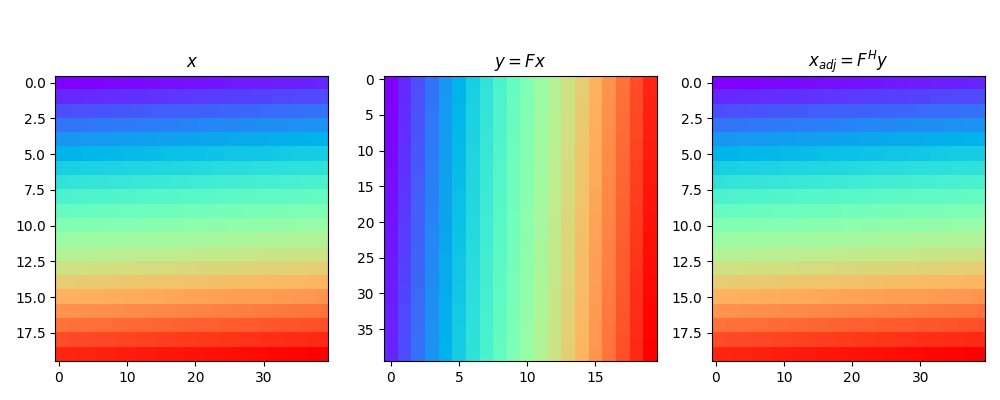# Transpose#

This example shows how to use the `pylops.Transpose` operator. For arrays that are 2-dimensional in nature this operator simply transposes rows and columns. For multi-dimensional arrays, this operator can be used to permute dimensions

```import matplotlib.pyplot as plt
import numpy as np

import pylops

plt.close("all")
np.random.seed(0)
```

Let’s start by creating a 2-dimensional array

```dims = (20, 40)
x = np.arange(800).reshape(dims)
```

We use now the `pylops.Transpose` operator to swap the two dimensions. As you will see the adjoint of this operator brings the data back to its original model, or in other words the adjoint operator is equal in this case to the inverse operator.

```Top = pylops.Transpose(dims=dims, axes=(1, 0))

y = Top * x

fig, axs = plt.subplots(1, 3, figsize=(10, 4))
fig.suptitle("Transpose for 2d data", fontsize=14, fontweight="bold", y=1.15)
axs.imshow(x, cmap="rainbow", vmin=0, vmax=800)
axs.set_title(r"\$x\$")
axs.axis("tight")
axs.imshow(y, cmap="rainbow", vmin=0, vmax=800)
axs.set_title(r"\$y = F x\$")
axs.axis("tight")